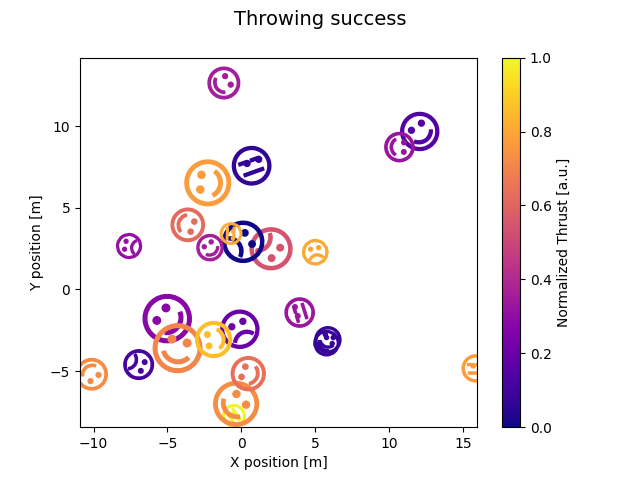# Mapping marker properties to multivariate data#

This example shows how to use different properties of markers to plot multivariate datasets. Here we represent a successful baseball throw as a smiley face with marker size mapped to the skill of thrower, marker rotation to the take-off angle, and thrust to the marker color.import numpy as np
import matplotlib.pyplot as plt
from matplotlib.markers import MarkerStyle
from matplotlib.transforms import Affine2D
from matplotlib.text import TextPath
from matplotlib.colors import Normalize

SUCCESS_SYMBOLS = [
TextPath((0, 0), "☹"),
TextPath((0, 0), "😒"),
TextPath((0, 0), "☺"),
]

N = 25
np.random.seed(42)
skills = np.random.uniform(5, 80, size=N) * 0.1 + 5
takeoff_angles = np.random.normal(0, 90, N)
thrusts = np.random.uniform(size=N)
successful = np.random.randint(0, 3, size=N)
positions = np.random.normal(size=(N, 2)) * 5
data = zip(skills, takeoff_angles, thrusts, successful, positions)

cmap = plt.colormaps["plasma"]
fig, ax = plt.subplots()
fig.suptitle("Throwing success", size=14)
for skill, takeoff, thrust, mood, pos in data:
t = Affine2D().scale(skill).rotate_deg(takeoff)
m = MarkerStyle(SUCCESS_SYMBOLS[mood], transform=t)
ax.plot(pos, pos, marker=m, color=cmap(thrust))
fig.colorbar(plt.cm.ScalarMappable(norm=Normalize(0, 1), cmap=cmap),
ax=ax, label="Normalized Thrust [a.u.]")
ax.set_xlabel("X position [m]")
ax.set_ylabel("Y position [m]")

plt.show()


Gallery generated by Sphinx-Gallery